2020年12月6日 作者 老王

# 1 引言

①使用LineRenderer
②代码生成网格画虚线
③使用片元着色器画虚线
④使用几何着色器画虚线
⑤使用UILineRenderer
⑥使用Vectrosity插件
6种方式都不是完美的，有利有弊，需要根据实际项目进行选择与调整。

# 2 LineRenderer画虚线①添加LineRenderer组件 Component 》Effects》LineRenderer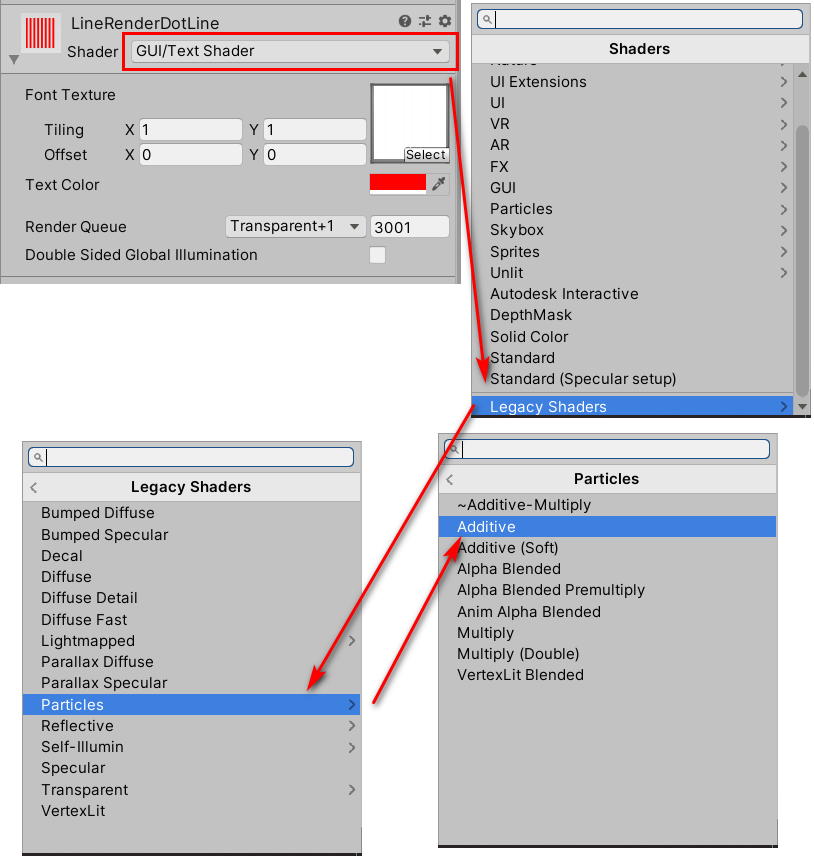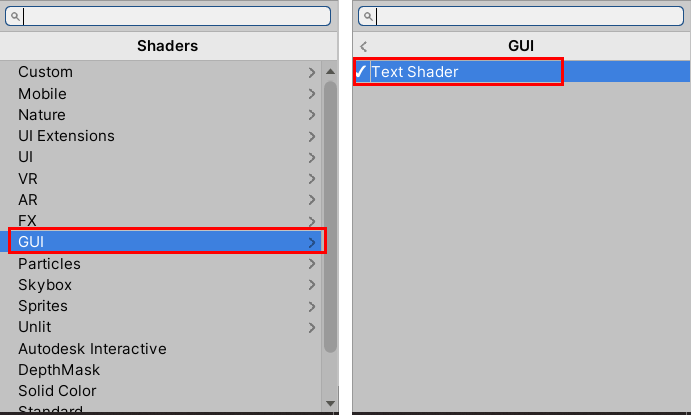③将②创建的材质球指定给LineRenderer，设置Positions并调节其他参数，这里就不细说了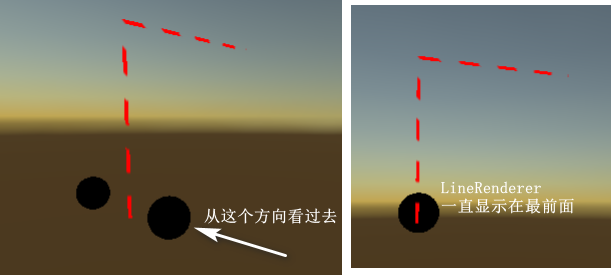Unity会先渲染不透明物体(开启了深度测试与深度写入)，然后再渲染半透明物体(一般会开启深度测试，但关闭深度写入)。

Shader "GUI/3D Text Shader" {
Properties {
_MainTex ("Font Texture", 2D) = "white" {}
_Color ("Text Color", Color) = (1,1,1,1)
}

Tags { "Queue"="Transparent" "IgnoreProjector"="True" "RenderType"="Transparent" }
Lighting Off Cull Off ZWrite Off Fog { Mode Off }
Blend SrcAlpha OneMinusSrcAlpha
Pass {
Color [_Color]
SetTexture [_MainTex] {
combine primary, texture * primary
}
}
}
}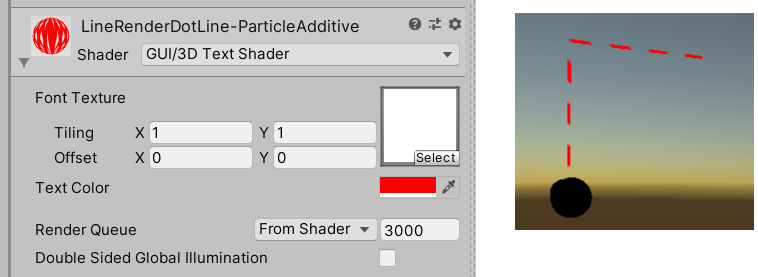②若想在UGUI上使用LineRenderer画虚线该怎么处理？
Camera的渲染模式一定要选择为Screen Space – Camera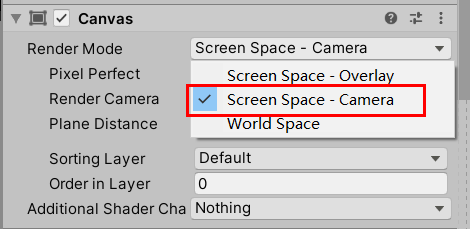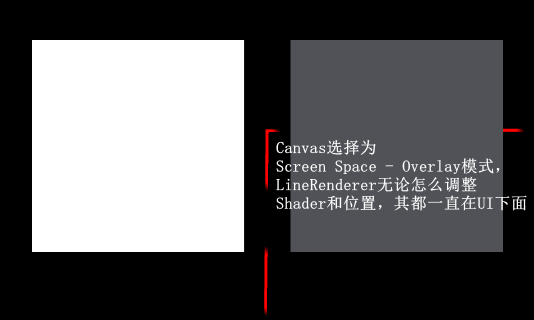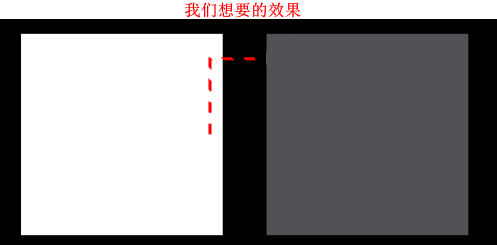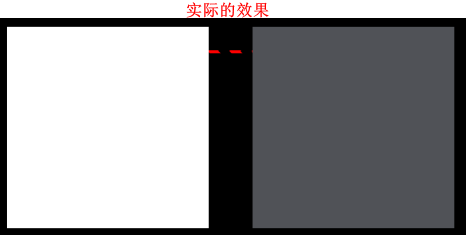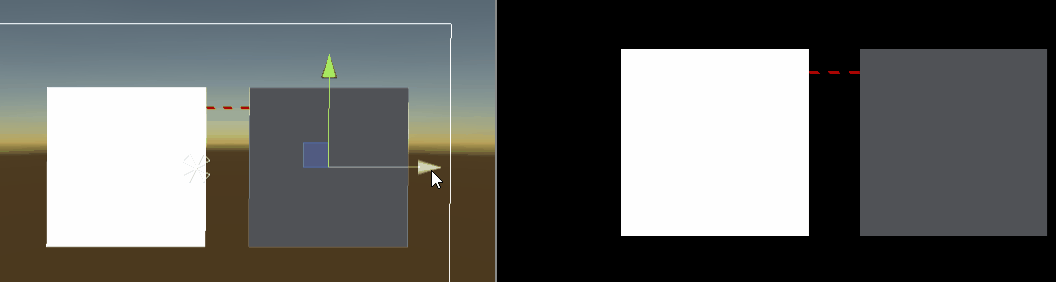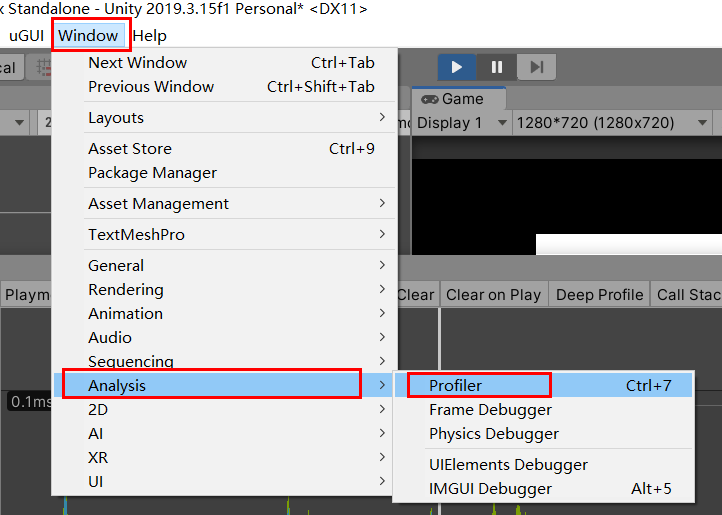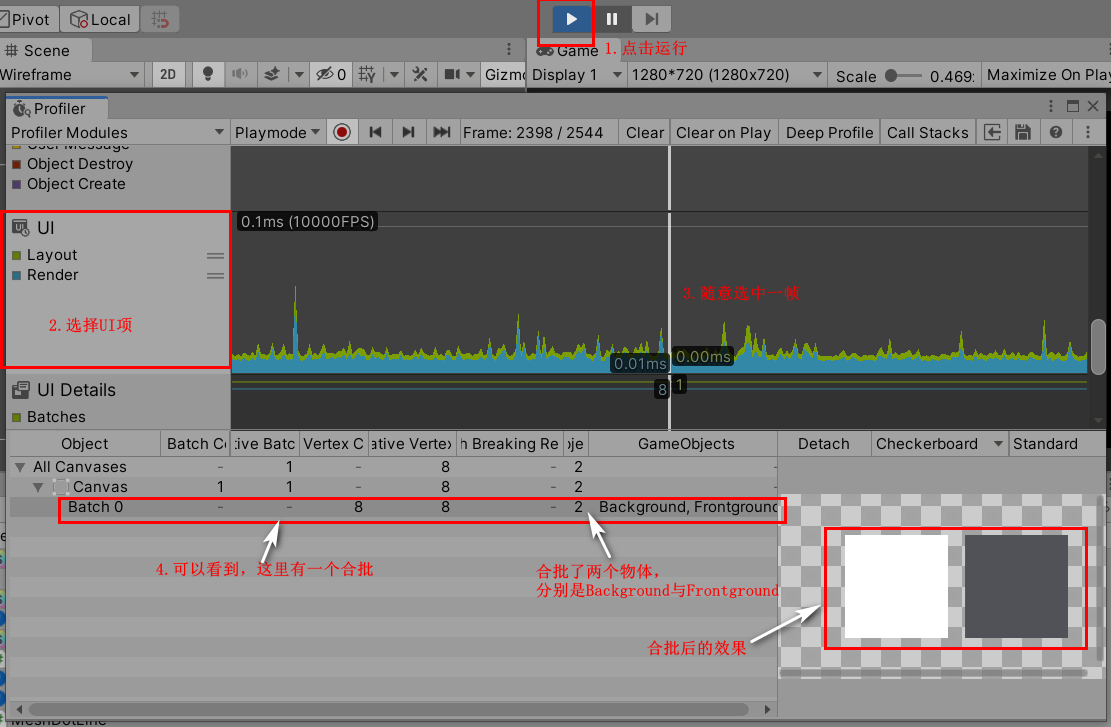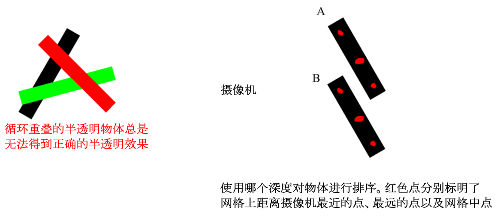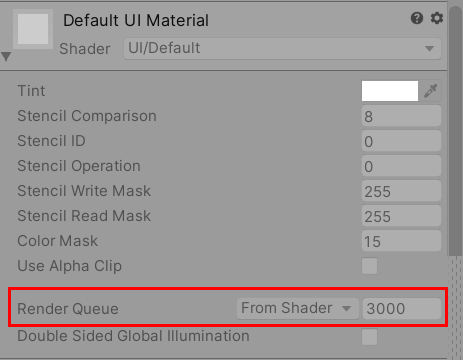ps：Unity提前定义的5个渲染队列如下
| 名称 | 队列索引号 | 描述 |
|–|–|–|
| Background | 1000 | 在其他任何渲染队列之前被渲染，正如名称一样，一般用来渲染背景物体 |
| Geometry | 2000| 默认的渲染队列，不透明物体使用此渲染队列 |
| AplhaTest | 2450| 需要进行透明度测试的物体使用此物体，从Geometry抽离出来，原因是在所有不透明物体渲染完成后再渲染它们更高效 |
| Transparent | 3000 | 此队列的物体会在前面三个渲染队列的物体渲染后，按照物体从后往前的顺序进行渲染。任何使用了透明度混合的物体都应该使用这个渲染队列 |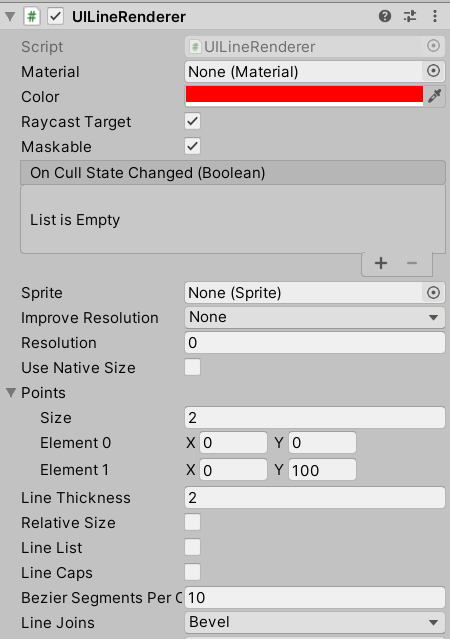# 3 代码生成网格画虚线

Mesh这个类该怎么使用，可以参考我之前写的《Unity3D C#数学系列之创建圆柱体》。

using System.Collections.Generic;
using UnityEngine;

public class MeshDotLine : MonoBehaviour
{
private void Start()
{
MeshFilter mf = gameObject.GetComponent<MeshFilter>();
if (mf == null)
{
}
MeshRenderer mr = gameObject.GetComponent<MeshRenderer>();
if (mr == null)
{
}
Mesh mesh = new Mesh();
mf.mesh = mesh;
mr.material.SetColor("_Color", Color.yellow);
CreateDotLineMesh(mesh, 100, 0.5f, 20);
}

/// <summary>
/// 生成虚线网格.
/// </summary>
/// <param name="mesh">网格</param>
/// <param name="length">总长度</param>
/// <param name="cnt">虚线段数</param>
private void CreateDotLineMesh(Mesh mesh, float length, float ratio, int cnt)
{
List<Vector3> vertices = new List<Vector3>();

List<int> indices = new List<int>();
List<Vector2> uvs = new List<Vector2>();

float deltaLength = length / cnt;
float solidLength = deltaLength * ratio;
float deltaUv = 1.0f / cnt;
float solidUv = deltaUv * ratio;
for (int i = 0; i < cnt; i++)
{
float start = i * deltaLength;
vertices.Add(new Vector3(start + solidLength, 0, 0));

float startUv = i * deltaUv;
}
mesh.SetVertices(vertices);
mesh.SetIndices(indices, MeshTopology.Lines, 0);
}
}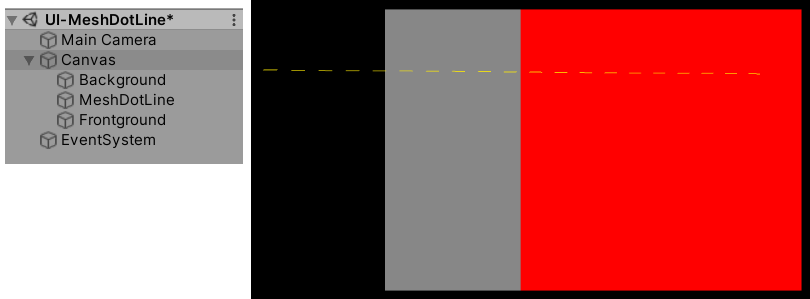# 4 使用片元着色器画虚线

## 4.1 源码

Shader "Custom/UI/DotLine"
{
Properties
{
_Color ("Tint", Color) = (1,1,1,1)
_Cnt ("Cnt", float) = 100
_Ratio ("Ratio", Range(0, 1.0)) = 0.5
[Toggle(VERTICAL)] _Y ("Y？", float) = 0
}

{
Tags
{
"Queue"="Transparent"
"IgnoreProjector"="True"
"RenderType"="Transparent"
"PreviewType"="Plane"
"CanUseSpriteAtlas"="True"
}

Cull Off
Lighting Off
ZWrite Off
ZTest [unity_GUIZTestMode]
Blend One OneMinusSrcAlpha

Pass
{
Name "Default"
CGPROGRAM
#pragma vertex vert
#pragma fragment frag
#pragma target 2.0
#pragma multi_compile __ VERTICAL

#include "UnityCG.cginc"
#include "UnityUI.cginc"

struct appdata_t
{
float4 vertex   : POSITION;
float2 texcoord : TEXCOORD0;
};

struct v2f
{
float4 vertex   : SV_POSITION;
float2 texcoord  : TEXCOORD0;
};

fixed4 _Color;
float _Cnt;
fixed _Ratio;

v2f vert(appdata_t v)
{
v2f OUT;
OUT.vertex = UnityObjectToClipPos(v.vertex);
OUT.texcoord = v.texcoord;
return OUT;
}

fixed4 frag(v2f IN) : SV_Target
{
fixed4 color = _Color;

#if VERTICAL

float y = IN.texcoord.y * _Cnt;
int intY = int(y);
color.a *= step(y-intY, _Ratio);

#else

float x = IN.texcoord.x * _Cnt;
int intX = int(x);
color.a *= step(x-intX, _Ratio);

#endif

color.rgb *= color.a;
return color;
}
ENDCG
}
}
}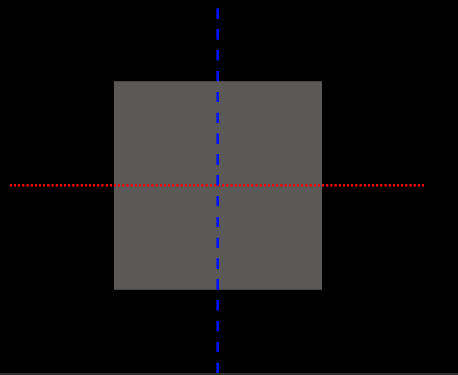float x = IN.texcoord.x * _Cnt;
int intX = int(x);
color.a *= step(x-intX, _Ratio);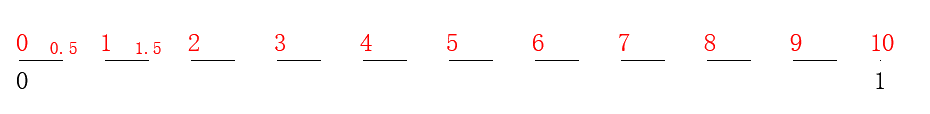## 4.2 MaterialPropertyDrawer

[Toggle(VERTICAL)] _Y ("Y？", float) = 0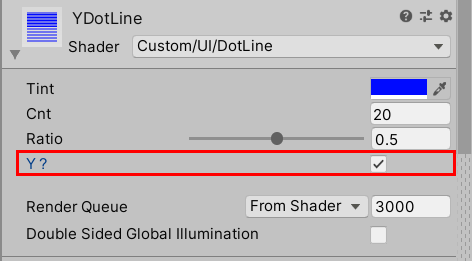// 勾选后会默认定义 _Y_ON这个keyword
[Toggle] _Y ("Y？", float) = 0


#pragma multi_compile __ VERTICAL


#pragma multi_compile可以实现只写一份代码但可由用户选择不同功能的目的。Untiy其实是在背后帮我们生成了几份不同的Shader，这写不同的Shader称为Shader变种（shader variants）。咱们可以通过选中Shader并打开Compiled code查看此Shader有几种Shader变种。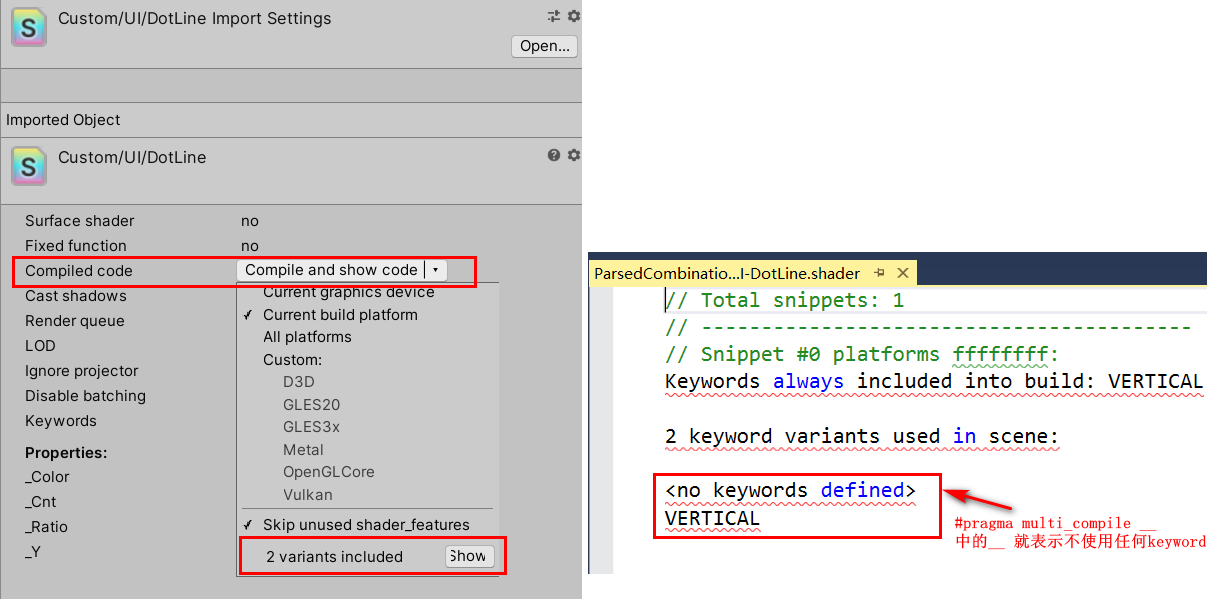#pragma shader_feature ENABLE_FANCY
#pragma multi_compile __ ENABLE_FANCY


## 4.3 unity_GUIZTestMode

unity_GUIZTestMode会由Canvas自动设置。

ps: ZTest 可取值为：Greater , GEqual , Less , LEqual , Equal , NotEqual , Always , Never , Off，默认是 LEqual，ZTest Off 等同于 ZTest Always。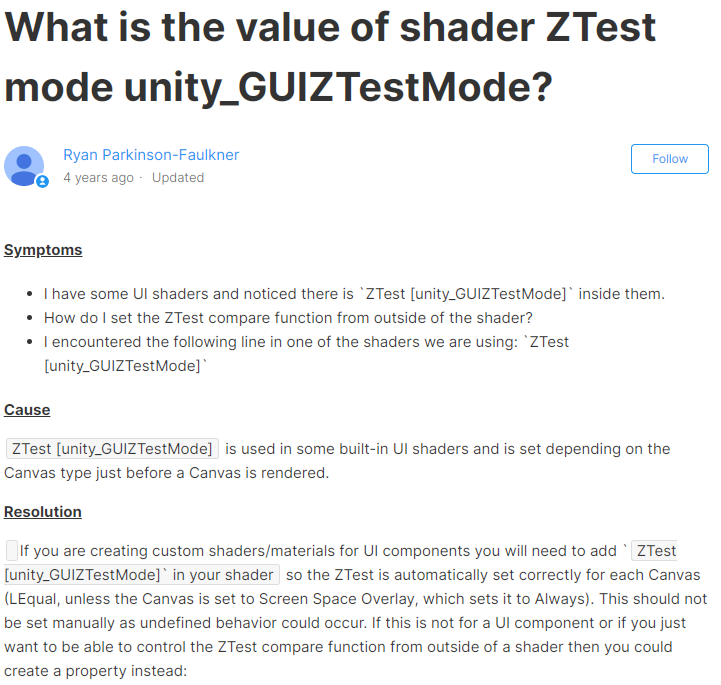# 5 使用几何着色器画虚线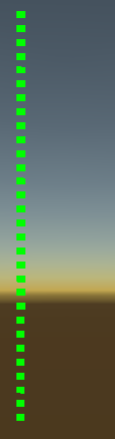using UnityEngine;

public class GeoDotLineDemo : MonoBehaviour
{
void Start()
{
MeshFilter mf = gameObject.GetComponent<MeshFilter>();
if (mf == null)
{
}
MeshRenderer mr = gameObject.GetComponent<MeshRenderer>();
if (mr == null)
{
}
Mesh mesh = new Mesh();
mf.mesh = mesh;

mesh.vertices = new Vector3[]
{
new Vector3(0, 0, 0),
new Vector3(0, 100, 0),
};
mesh.SetIndices(new int[]{0, 1}, MeshTopology.LineStrip, 0);
mesh.uv = new Vector2[]
{
Vector2.zero,
new Vector2(1, 1),
};
}

}


Shader "Custom/Geometry/GeoDotLine"
{
Properties
{
_MainTex ("Main Tex", 2D) = "white" {}
_LineColor ("Line Color", color) = (0, 1, 0, 1)
_LineWidth ("Line Width", float) = 2
_Cnt ("Cnt", float) = 30
_Ratio ("Ratio", Range(0, 1.0)) = 0.5
}

{
Cull Off
Blend SrcAlpha OneMinusSrcAlpha
Tags {"Queue" = "Transparent"}

LOD 100
Pass
{
CGPROGRAM
#pragma target 4.0
#pragma vertex vert
#pragma geometry geo
#pragma fragment frag

#include "UnityCG.cginc"

struct appdata
{
float4 vertex : POSITION;
float2 uv : TEXCOORD0;
};

struct v2f
{
float2 uv : TEXCOORD0;
float4 vertex : SV_POSITION;
};

sampler2D _MainTex;
float4 _MainTex_ST;
fixed4 _LineColor;
float _LineWidth;
float _Cnt;
float _Ratio;

v2f vert (appdata v)
{
v2f o;
o.vertex = v.vertex;
o.uv = v.uv;
return o;
}

//产生最大的顶点数，append到triStream的次数应该小于此。最大好像是64
[maxvertexcount(6)]
void geo(line appdata l, inout TriangleStream<v2f> triStream)
{
v2f pIn;
v2f v;                               //  先生成四个顶点，构造两个三角形

float4 a = l.vertex;
float4 b = l.vertex;

float halfLineWidth =  0.5 * _LineWidth;

v.vertex = UnityObjectToClipPos(a + float4(halfLineWidth, 0, 0, 0));
v.uv = float2(0.0f, 1.0f);
v.vertex = UnityObjectToClipPos(b + float4(halfLineWidth, 0, 0, 0));
v.uv = float2(1.0f, 1.0f);
v.vertex = UnityObjectToClipPos(b + float4(-halfLineWidth, 0, 0, 0));
v.uv = float2(1.0f, 0.0f);
v.vertex = UnityObjectToClipPos(a + float4(-halfLineWidth, 0, 0, 0));
v.uv = float2(0.0f, 0.0f);

triStream.Append(v);
triStream.Append(v);
triStream.Append(v);
triStream.RestartStrip();           // 重置三角形计数，提交三角形
triStream.Append(v);
triStream.Append(v);
triStream.Append(v);
triStream.RestartStrip();
}

fixed4 frag (v2f i) : SV_Target
{
fixed4 color = _LineColor;
float x = i.uv.x * _Cnt;
int intX = int(x);
color.a *= step(x-intX, _Ratio);
return color;
}
ENDCG
}
}
}


# 6 其他方式

⑤使用UILineRenderer
⑥使用Vectrosity插件

UILineRenderer来源于unity-ui-extensions，里面包含了很多其他控件，可以参考学习。

Vectrosity插件